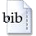User: GuestLogin
Author(s):
Tobias Neckel, Alfredo Parra Hinojosa, Florian Rupp
Title:
Path-Wise Algorithms for Random & Stochastic ODEs with Applications to Ground-Motion-Induced Excitations of Multi-Storey Buildings
Abstract:
Random effects play a vital role in various physical phenomena and have to be addressed in the mathematical modeling and numerical simulations of such processes. In this contribution, the concepts of random (ordinary) differential equations (RODEs) and stochastic (ordinary) differential equations (SODEs) are reviewed. Details concerning the numerical solution of problems formulated via SODEs and RODEs are summarised. In particular, key features of the averaged Euler and Heun as well as K-RODE...    »
Keywords:
random differential equation, stochastic differential equation, path-wise solution, numerical methods, Kanai-Tajimi earthquake model
Year:
2017
Language:
en
DOI:
doi:10.14459/2017md1350560BibTeX